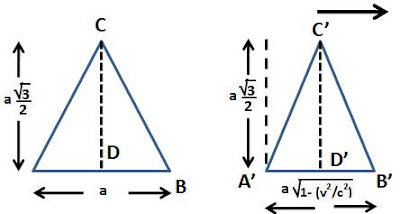## Sunday, September 13, 2009

### Irodov Problem 1.341

a) Let ABC be the original triangle. Since all its sides are equal to a it is an equilateral triangle. Let CD be the perpendicular bisector along which the triangle moves with a velocity v w.r.t to frame K. From elementary trigonometry.

Let A'B'C' be the triangle as seen from a frame K. w.r.t to K, the triangle will shrink by a factoralong the direction of motion (along CD). However, along the direction perpendicular to the motion of the triangle (along AB) all lengths will remain intact.

In other words,
AB = A'B' = a, AD = A'D' = a/2, C'D' would Thus, w.r.t to frame K, the triangle will no longer be equilateral since the sides A'C and C'B' would have shrunk. Hence, we have,b) In this part the triangle is moving in the direction of one of the sides (along AB) and not the bisector. Thus, all measurements of the triangle in this direction shrink by a factorrelative to frame K. The measurements in the perpendicular direction (along CD) however remain intact.

Thus, we have,